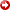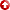--> Integrating Probabilistic Neural Networks and Generalized Boosted Regression Modelling for Lithofacies Classifications and Formation Permeability Estimation
[First Hit]# Integrating Probabilistic Neural Networks and Generalized Boosted Regression Modelling for Lithofacies Classifications and Formation PermeabilityEstimation## Abstract

Multiscale modelling of formation permeability is a crucial step in overall reservoir characterization. ITherefore, an integrated procedure was adopted for accurate Lithofacies classification prediction to be incorporated with well log attributes into core permeability modeling. Probabilistic Neural Networks (PNN) and Generalized Boosted Regression Modeling (GBM) were adopted for Efficient Lithofacies Classification and Formation PermeabilityEstimation, respectively. PNN is an implementation of a statistical algorithm called kernel discriminant analysis in which the operations are organized into a multi-layered feedforward network with four layers: Input, Pattern, Summation, and Output layers. It was used to model Lithofacies sequences to predict discrete lithofacies distribution at missing intervals. Then, GBM was used as a to build a nonlinear relationship between core and log data. GBM is a recent data mining technique that has shown considerable success in predictive accuracy as it maintains a monotonic relationship between the response and each predictor. The well log interpretations that were adopted are neutron porosity, shale volume, and water saturation given depth; however, the measured discrete lithotypes are Sand, Shaly Sand, and Shale. Firstly, PNN was adopted for modelling and prediction discrete Lithofacies distribution at missing intervals. The classified Lithofacies were considered as a discrete independent variable in core permeability modelling in order to provide different model fits given each Lithofacies type to capture the permeability variation. Then, GBM was applied to build the statistical modelling and create the relationship between core permeability and the explanatory variables of well logs and Lithofacies. In GBM results, Root Mean Square Prediction Error (RMSPE) and adjusted R-square have incredible positive values, as there was an excellent matching between the measured and predicted core permeability. GBM has led to overcome the multicollinearity that was available between one pair of the predictors. Based on the same dataset, the PNN Lithofacies algorithm is the best classification approach as the total percent correct of the predicted discrete Lithofacies has exceeded 97.5% in comparison with other methods such as Linear Discriminant Analysis and Support Vector Machine. In addition, the RMSPE and Adjusted R-square obtained by GBM are much better than linear regression methods that has been applied on the same data before.